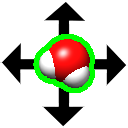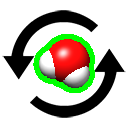### Tool: Fit in Map

Fit in Map locally optimizes the fit of atomic coordinates into a density map or one density map into another. The maps usually represent electron density, but other types of volume data can also be used.

The command implementation, fitmap, also includes some important features not available in the Fit in Map dialog:

### Dialog and Basic Usage

Fit in Map can be started from the Volume Data section of the Tools menu (more...).

Fit [ fit-model ] in map [ ref-model ]

The fit-model is the set of atoms or a map (volume data) to be fit into the ref-model map. Each should be chosen from the pulldown menu of models already open in ChimeraX. When atoms are fit, an entire atomic model or just the currently selected atoms can be used. If an entire atomic model is chosen, any parts not desired for fitting (such as solvent, ligands, or extra chains) should be deleted from that model.

Since the optimization is local rather than global, the fit model should be placed in a trial position relative to the reference map before fitting. This usually involves interactive manipulation with mouse modes that move only the selected model(s)or the model that was clicked. See selective manipulation.

Only the current display region (which may be a cropped rectangular box) of the ref-model map is used. All of the grid points in the region are used at full resolution, regardless of what step level and threshold (contour level) are used to display the ref-model map. However, for map-in-map fitting, the fit-model map data are restricted by its step level, display region, and optionally its lowest threshold level.

Clicking Fit performs local optimization of the fit (atoms-in-map or map-in-map) using the current options. The calculation will stop and the fit model will be repositioned after the earliest of:

The goodness-of-fit values Correlation and/or Average map value are reported in the dialog. Clicking Update gives the current fit values without performing any fitting.

The fit values, number of steps taken, and fit model displacement (translational shift and angle of rotation) are reported in the Log. The transformation of the fit model relative to the reference map is described with a transformation matrix in which the first three columns of the matrix describe a rotation and the fourth describes a translation (performed after the rotation). The transformation is also described as an axis of rotation (a unit vector), point on the axis, degrees of rotation, and shift parallel to the axis.

Clicking Fit again may further improve the results, especially if convergence was not reached. Undo backtracks through transformations of the fit model relative to the reference map applied by Fit in Map, whereas Redo reapplies them.

The clashes command with continuous true can be used to monitor for close contacts between atomic structures as they are moved, and atomic models can be saved in their positions relative to the reference map.

Options:

• Real-time correlation / average update – update fit values continuously as a model is moved
• Use map simulated from atoms, resolution [r] – generate a density map from the fit model atomic coordinates and use map-in-map fitting (but report both atoms-in-map and map-in-map fit values). The map is generated by describing each atom as a Gaussian distribution of width proportional to r and amplitude proportional to the atomic number (other parameters set to molmap defaults).
• Use only data above contour level from first map – exclude data outside the fit map contour surface from map-in-map fitting, i.e., exclude data values below the map's lowest threshold level
• whether to Optimize the overlap or the correlation during map-in-map fitting
• Correlation calculated about mean data value – whether the mean values or zero (default) should be used to calculate the correlation during map-in-map fitting
• whether to Allow fit model rotation and/or shift movements
• Move whole molecules – when selected atoms are specified as the fit-model, whether to move just those atoms or the entire atomic model(s) containing them. Either way, only the selected atoms will be used to calculate the fit.

Atoms-in-map fitting:
The average map value at fit atom positions is maximized. For each atom within the bounds of the reference map, the map value is found by trilinear interpolation from the eight corners of the enclosing data grid cell. Atoms outside the bounds of the map are not used for computing averages.
Map-in-map fitting:
Either the overlap or the correlation can be maximized. The calculation can include all nonzero-valued fit map grid points or only those with values above the map's lowest threshold level (see options). The overlap is the sum over fit map grid points of the product of the fit map value and the reference map value at that point, determined by trilinear interpolation. It can be expressed as the inner product of vectors u and v containing the fit map values and the corresponding interpolated reference map values:
overlap = <u,v>
In similar notation,
 correlation = | u || v |
or if the correlation about mean option is turned on,
 correlation = |u–uave||v–vave|
where uave is a vector with all components equal to the average of the components of u and vave is defined analogously. The correlation equals the cosine of the angle between the vectors (after subtraction of averages) and can range from –1 to 1, whereas the range of overlap values depends on the scaling of the maps.

Close closes the Fit in Map dialog.

### Notes

Local optimization algorithm. If rotation and translation are both allowed, every even step is a translation and every odd step is a rotation. The center of rotation is the geometric center of the fit atoms or fit map grid points, whichever applies. Optimization is by steepest ascent. Map value gradients at atom positions or fit map points are calculated using trilinear interpolation of the gradients at the reference map points. Gradients at grid points are calculated by the center difference method. Atoms or fit map points outside the reference map or within one voxel of the edge of the data at a given step do not contribute to the optimal direction at that step. The initial step size is the largest (default 0.5 grid unit, where a grid unit is the spacing between reference map grid points). If after four steps the maximum cumulative displacement is less than half the displacement achievable if all steps were in the same direction (e.g., half of 2.0 grid units = 1 grid unit), the step size is halved. Successive rounds of four steps with fixed step size and halving the step size based on the maximum displacement criterion are repeated until convergence (when step size falls below some minimum, default 0.01 grid unit) or the number of steps reaches some maximum (default 2000). Values other than the defaults can be specified with the fitmap command.

Comparing fits. Because local optima rather than a global optimum are found, it is often beneficial to explore and compare different fits. This can be done by opening multiple copies of the fit model and fitting them from different starting positions. The Model Panel can be used to hide and show individual copies.

Clipping and hiding are ignored. The calculation uses full maps even if partly hidden by clipping or zoning (with surface zone or volume zone).

Self-correlation ≠ 1. The correlation value of a map with itself in the same position should equal 1. However, in such cases, Fit in Map can report values much less than 1 because of floating-point rounding errors in grid point positions. Grid points at the boundary of a map can be found to lie outside the map, yielding interpolated values of 0.

Speed. Calculation time is proportional to the number of fit atoms or fit map grid points. Using a selection of protein α-carbons only, for example, will be substantially faster than using all atoms. The optimization calculation is written partly in C++ for speed and partly in Python.

UCSF Resource for Biocomputing, Visualization, and Informatics / February 2023